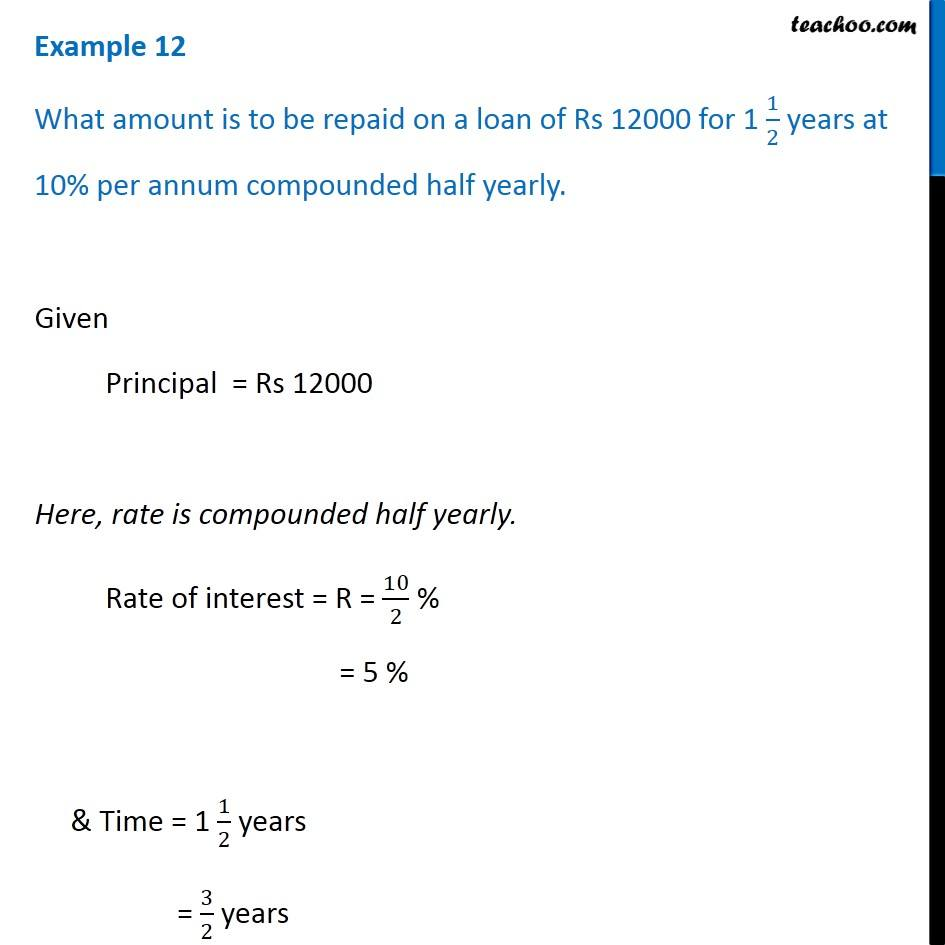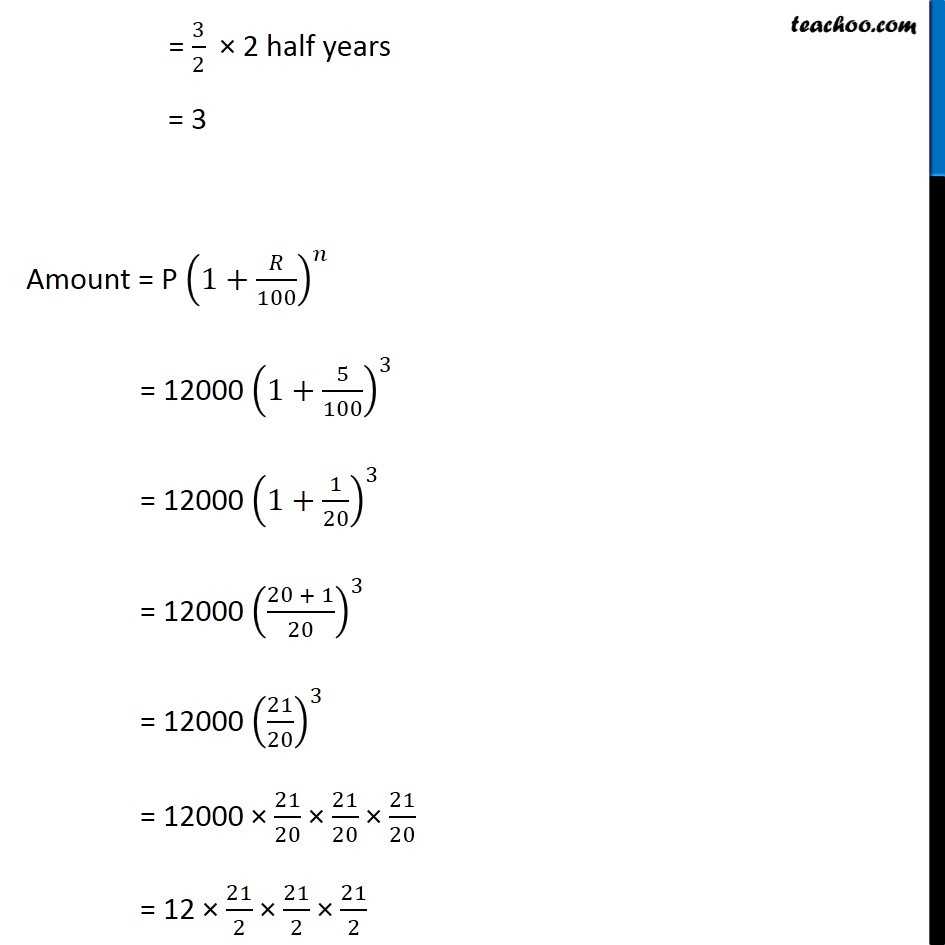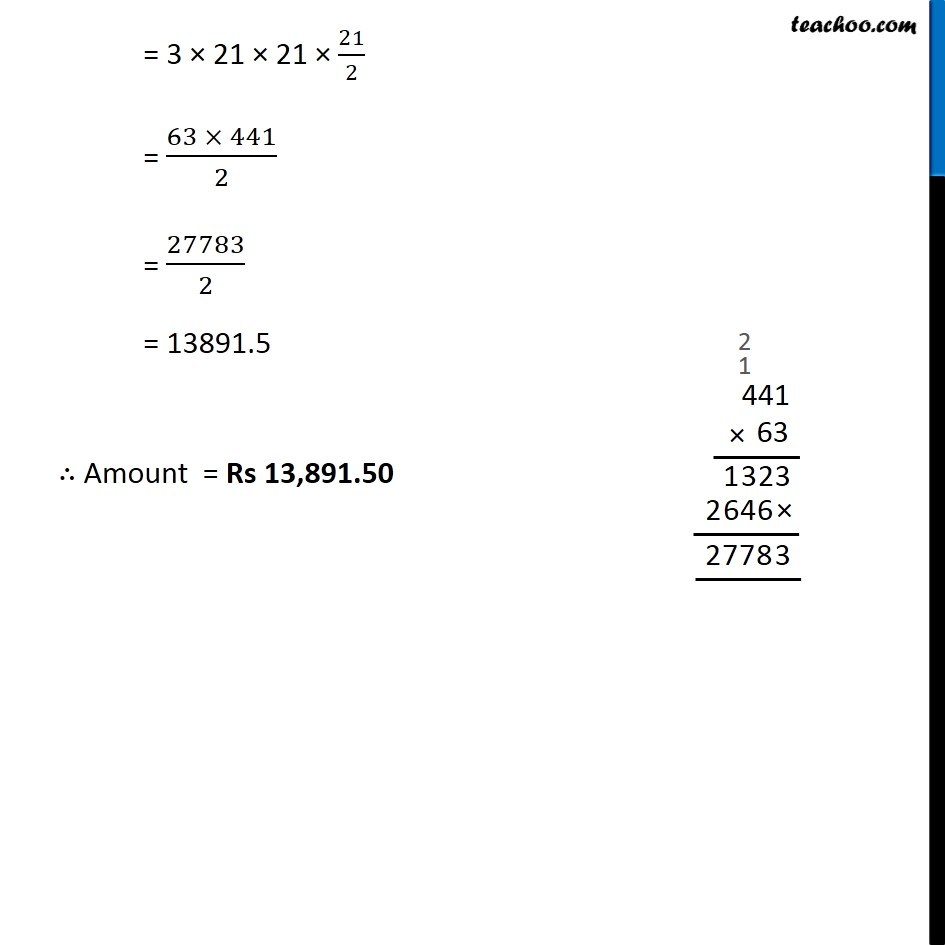Examples

Chapter 7 Class 8 Comparing Quantities
Serial order wiseLearn in your speed, with individual attention - Teachoo Maths 1-on-1 Class

### Transcript

Question 5 What amount is to be repaid on a loan of Rs 12000 for 1 1/2 years at 10% per annum compounded half yearly. Given Principal = Rs 12000 Here, rate is compounded half yearly. Rate of interest = R = 10/2 % = 5 % & Time = 1 1/2 years = 3/2 years = 3/2 × 2 half years = 3 Amount = P (1+𝑅/100)^𝑛 = 12000 (1+5/100)^3 = 12000 (1+1/20)^3 = 12000 ((20 + 1)/20)^3 = 12000 (21/20)^3 = 12000 × 21/20 × 21/20 × 21/20 = 12 × 21/2 × 21/2 × 21/2 = 3 × 21 × 21 × 21/2 = (63 × 441)/2 = 27783/2 = 13891.5 ∴ Amount = Rs 13,891.50